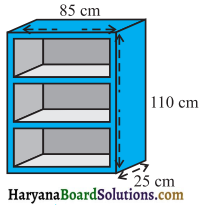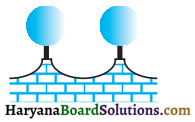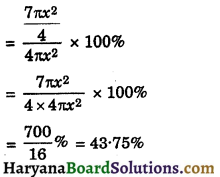# HBSE 9th Class Maths Solutions Chapter 13 Surface Areas and Volumes Ex 13.9

Haryana State Board HBSE 9th Class Maths Solutions Chapter 13 Surface Areas and Volumes Ex 13.9 Textbook Exercise Questions and Answers.

## Haryana Board 9th Class Maths Solutions Chapter 13 Surface Areas and Volumes Exercise 13.9

[This exercise is not from examination point of view]

Question 1.
A wooden bookshelf has external dimensions as follows: Height = 110 cm, Depth = 25 cm, Breadth = 85 cm (see Fig. 13.31). The thickness of the plank is 5 cm everywhere. The external faces are to be polished and the inner faces are to be painted. If the rate of polishing is 20 paise per cm2 and the rate of painting is 10 paise per cm2, find the total expenses required for polishing and painting the surface of the bookshelf.Solution:
We have
External dimensions of bookshelf are
Height = 110 cm, depth = 25 cm and breadth = 85 cm
Thickness of the plank = 5 cm
∴ Internal dimensions of bookshelf are Height = 110 – 4 × 5 = 90 cm
(there are 4 plank)
Breadth = 85 – 2 × 5 = 75 cm
Depth = 25 – 5 = 20 cm
Area to be polished = (110 × 85 + 2 × 110 × 25 + 2 × 85 × 25 + 4 × 75 × 5 + 2 × 110 × 5)
= 9350 + 5500 + 4250 + 1500 + 1100
= 21700 cm2
Rate of polishing = 20 paise/cm2
Cost of polishing = Rs. 21700 × $$\frac{20}{100}$$
= Rs. 4340
Area to be painted= 6 × 75 × 20 + 2 × 90 × 20 + 75 × 90
= 9000 + 3600 + 6750
= 19350 cm2
Cost of painting = 10 paise/cm2
Cost of painting = Rs. $$\frac{19350 \times 10}{100}$$
= Rs. 1935
Total expenses = Rs. 4340 + Rs. 1935
= Rs. 6275
Hence, total expenses = Rs. 6275.Question 2.
The front compound wall of a house is decorated by wooden spheres of diameter 21 cm, placed on small supports as shown in Fig. 13.32. Eight such spheres are used for this purpose, and are to be painted silver. Each support is a cylinder of radius 1.5 cm and height 7 cm and is to be painted black. Find the cost of paint required if silver paint costs 25 paise per cm2 and black paint costs 5 paise per cm2.Fig. 13.32
Solution:
We have,
Diameter of sphere = 21 cm
∴ Radius of sphere (R) = $$\frac{21}{2}$$ = 10.5 cm
Radius of cylinder (r) = 1.5 cm
Height of cylinder (h) = 7 cm
Surface area for silver paint = 8(C.S.A. of the sphere – base area of cylinder on which sphere is resting)
= 8(4πR2 – πr2)
= 8π(4R2 – r2)
= 8π[4 × (10.5)2 – (1.5)2]
= 8 × $$\frac{22}{7}$$[4 × 110.25 – 2.25]
= 8 × $$\frac{22}{7}$$[438.75]
= 11031.43 cm2 (approx.)
Rate of silver paint = 25 paise/cm2
Cost of silver paint = Rs. $$\frac{11031.43 \times 25}{100}$$
= Rs. 2757.86 (approx.)
Surface area to be black painted = 8 C.S.A. of cylinder
= 8 × 2πrh
= 8 × 2 × $$\frac{22}{7}$$ × 1.5 × 7
= 528 cm2
Rate of black paint = 5 paise/cm2
Cost of black paint = Rs. $$\frac{528 \times 5}{100}$$ = Rs. 26.4
Total cost of painting = 2757.86 + 26.4
= Rs. 2784.26
Hence, total cost of painting = Rs. 2784.26.Question 3.
The diameter of a sphere is decreased by 25%. By what percent does its curved surface area decrease?
Solution:
Let the diameter of sphere be 2x.
∴ Radius of the sphere (r1) = x
Curved surface area of sphere = 4πx2
After decreasing its diameter by 25%, then
New diameter = 2x – 25% of 2x
= $$2 x-\frac{25}{100} \times 2 x$$
= $$2 x-\frac{x}{2}=\frac{3 x}{2}$$
New radius (r2) = $$\frac{3 x}{4}$$
Curved surface area of new sphere
= $$4 \pi r_2^2=4 \pi \times\left(\frac{3 x}{4}\right)^2$$
= $$\frac{4 \pi \times 9 x^2}{16}=\frac{9 \pi x^2}{4}$$
Decrease in curved surface area
= $$4 \pi x^2-\frac{9 \pi x^2}{4}$$
= $$\frac{16 \pi x^2-9 \pi x^2}{4}$$
= $$\frac{7 \pi x^2}{4}$$
Decrease % in curved surface areaHence, decrease % in curved surface area = 43.75%.